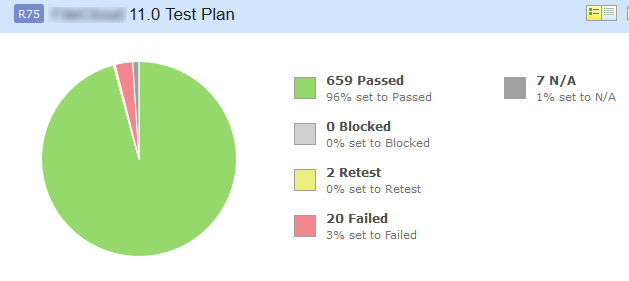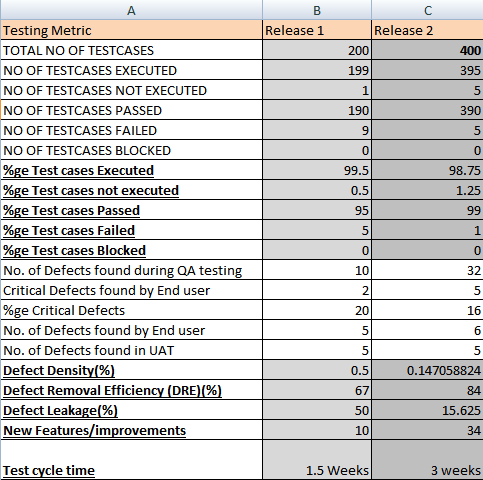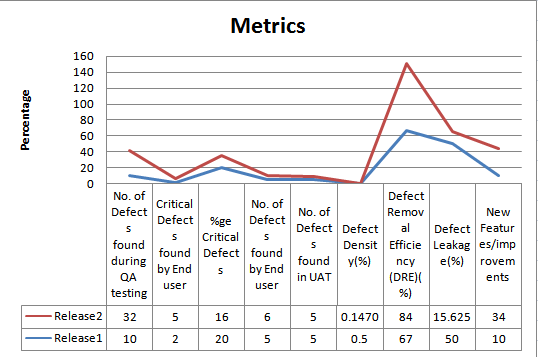# Software test metrics how it helps?

January 15, 2016

“We can’t control things which we can’t measure” Software Test Metrics are used to, Take the decision for next phase of activities such as, estimate the cost & schedule of future projects. Understand the kind of improvement required to success the project Take decision on process or technology to be modified etc Software Test Metrics […]

“We can’t control things which we can’t measure”

Software Test Metrics are used to,

• Take the decision for next phase of activities such as, estimate the cost & schedule of future projects.
• Understand the kind of improvement required to success the project
• Take decision on process or technology to be modified etc

Software Test Metrics

Software Metrics are used to measure the quality of the project. Simply, Metric is a unit used for describing an attribute. Metric is a scale for measurement.

Test metrics example:

• How many defects are existed within the module?
• How many test cases are executed per person?
• What is the Test coverage %?

Why Test Metrics?

Generation of Software Test Metrics is the most important responsibility of the Software Test Lead/Manager.

Test Metrics are used to,

1. Take the decision for next phase of activities such as, estimate the cost & schedule of future projects.
2. Understand the kind of improvement required to success the project
3. Take decision on process or technology to be modified etc.

Type of metrics
Base Metrics (Direct Measure)

Base metrics constitute the raw data gathered by a Test Analyst throughout the testing effort. These metrics are used to provide project status reports to the Test Lead and Project Manager; they also feed into the formulas used to derive Calculated Metrics.
Ex: # of Test Cases, # of Test Cases Executed

Calculated Metrics (Indirect Measure)

Calculated Metrics convert the Base Metrics data into more useful information. These types of metrics are generally the responsibility of the Test Lead and can be tracked at many different levels (by module, tester, or project).
Ex: % Complete, % Test Coverage

## Definitions and Formulas for Calculating Metrics:

#1) %ge Test cases Executed: This metric is used to obtain the execution status of the test cases in terms of %ge.

%ge Test cases Executed = (No. of Test cases executed / Total no. of Test cases written) * 100.

So, from the above data,
%ge Test cases Executed = (65 / 100) * 100 = 65%

#2) %ge Test cases not executed: This metric is used to obtain the pending execution status of the test cases in terms of %ge.

%ge Test cases not executed = (No. of Test cases not executed / Total no. of Test cases written) * 100.

So, from the above data,
%ge Test cases Blocked = (35 / 100) * 100 = 35%

#3) %ge Test cases Passed: This metric is used to obtain the Pass %ge of the executed test cases.

%ge Test cases Passed = (No. of Test cases Passed / Total no. of Test cases Executed) * 100.

So, from the above data,
%ge Test cases Passed = (30 / 65) * 100 = 46%

#4) %ge Test cases Failed: This metric is used to obtain the Fail %ge of the executed test cases.

%ge Test cases Failed = (No. of Test cases Failed / Total no. of Test cases Executed) * 100.

So, from the above data,
%ge Test cases Passed = (26 / 65) * 100 = 40%

#5) %ge Test cases Blocked: This metric is used to obtain the blocked %ge of the executed test cases. A detailed report can be submitted by specifying the actual reason of blocking the test cases.

%ge Test cases Blocked = (No. of Test cases Blocked / Total no. of Test cases Executed) * 100.

So, from the above data,
%ge Test cases Blocked = (9 / 65) * 100 = 14%#6) Defect Density = No. of Defects identified / size

(Here “Size” is considered as requirement. Hence here the Defect Density is calculated as number of defects identified per requirement. Similarly, Defect Density can be calculated as number of Defects identified per 100 lines of code [OR] No. of defects identified per module etc.)

So, from the above data,
Defect Density = (30 / 5) = 6

#7) Defect Removal Efficiency (DRE) = (No. of Defects found during QA testing / (No. of Defects found during QA testing +No. of Defects found by End user)) * 100

DRE is used to identify the test effectiveness of the system.
Suppose, During Development & QA testing, we have identified 100 defects.
After the QA testing, during Alpha & Beta testing, end user / client identified 40 defects, which could have been identified during QA testing phase.

Now, The DRE will be calculated as,
DRE = [100 / (100 + 40)] * 100 = [100 /140] * 100 = 71%

\$8) Defect Leakage: Defect Leakage is the Metric which is used to identify the efficiency of the QA testing i.e., how many defects are missed / slipped during the QA testing.

Defect Leakage = (No. of Defects found in UAT / No. of Defects found in QA testing.) * 100

Suppose, During Development & QA testing, we have identified 100 defects.
After the QA testing, during Alpha & Beta testing, end user / client identified 40 defects, which could have been identified during QA testing phase.

Defect Leakage = (40 /100) * 100 = 40%

#9) Defects by Priority: This metric is used to identify the no. of defects identified based on the Severity / Priority of the defect which is used to decide the quality of the software.

%ge Critical Defects = No. of Critical Defects identified / Total no. of Defects identified * 100
From the data available in the above table,
%ge Critical Defects = 6/ 30 * 100 = 20%

%ge High Defects = No. of High Defects identified / Total no. of Defects identified * 100
From the data available in the above table,
%ge High Defects = 10/ 30 * 100 = 33.33%

%ge Medium Defects = No. of Medium Defects identified / Total no. of Defects identified * 100
From the data available in the above table,
%ge Medium Defects = 6/ 30 * 100 = 20%

%ge Low Defects = No. of Low Defects identified / Total no. of Defects identified * 100
From the data available in the above table,
%ge Low Defects = 8/ 30 * 100 = 27%Improvements that can be taken in order to increase the quality of testing.

• Test data should be readily available in the testcase
• New Features should to be always tested first , to allow enough time for the developers to fix the bug
• Prioritizing test case while execution.
• Sanity test after bug fixes.